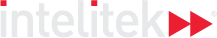### Course Overview

In the second course, the material expands to include logic and settheory, as well as an overview of the Pythagorean theorem and its applications which play an important role in advanced mathematics. There is a logical progression to the next lessons, which cover volume and surface area of basic shapes such as prisms, cylinders and spheres, as well as an introduction to exponents and their applications.

HOURS OF INSTRUCTION: 15

### Course Outline

• Logic
• Set Theory
• Pythagorean Theorem, Perimeter and Area of Polygons and Circles
• Volume and Surface Area
• Exponents